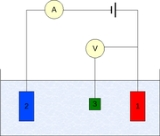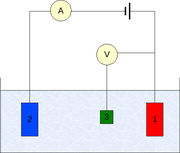xElectrode potentialEncyclopedia
Electrode potential, E, in electrochemistry
Electrochemistry
Electrochemistry is a branch of chemistry that studies chemical reactions which take place in a solution at the interface of an electron conductor and an ionic conductor , and which involve electron transfer between the electrode and the electrolyte or species in solution.If a chemical reaction is...

, according to an IUPAC definition, is the electromotive force
Electromotive force
In physics, electromotive force, emf , or electromotance refers to voltage generated by a battery or by the magnetic force according to Faraday's Law, which states that a time varying magnetic field will induce an electric current.It is important to note that the electromotive "force" is not a...

of a cell
Galvanic cell
A Galvanic cell, or Voltaic cell, named after Luigi Galvani, or Alessandro Volta respectively, is an electrochemical cell that derives electrical energy from spontaneous redox reaction taking place within the cell...

built of two electrode
Electrode
An electrode is an electrical conductor used to make contact with a nonmetallic part of a circuit...

s:
• on the left-hand side is the standard hydrogen electrode
Standard hydrogen electrode
The standard hydrogen electrode , is a redox electrode which forms the basis of the thermodynamic scale of oxidation-reduction potentials...

, and
• on the right-hand side is the electrode the potential of which is being defined.

By convention:
ECell := ECathodeEAnode

From the above, for the cell with the standard hydrogen electrode (potential of 0 by convention), one obtains:
ECell = ERight − 0 = EElectrode

The left-right convention is consistent with the international agreement that redox potentials be given for reactions written in the form of reduction half-reactions.

Electrode potential is measured in volt
Volt
The volt is the SI derived unit for electric potential, electric potential difference, and electromotive force. The volt is named in honor of the Italian physicist Alessandro Volta , who invented the voltaic pile, possibly the first chemical battery.- Definition :A single volt is defined as the...

s (V).

## MeasurementThe measurement is generally conducted using a three-electrode setup (see the drawing):
1. Working electrode
Working electrode
The working electrode is the electrode in an electrochemical system on which the reaction of interest is occurring. The working electrode is often used in conjunction with an auxiliary electrode, and a reference electrode in a three electrode system...

2. Counter electrode
Auxiliary electrode
The Auxiliary electrode, often also called the counter electrode, is an electrode used in a three electrode electrochemical cell for voltammetric analysis or other reactions in which an electrical current is expected to flow...

3. Reference electrode
Reference electrode
A reference electrode is an electrode which has a stable and well-known electrode potential. The high stability of the electrode potential is usually reached by employing a redox system with constant concentrations of each participants of the redox reaction.There are many ways reference...

(standard hydrogen electrode or an equivalent)

The measured potential of the working electrode may be either that at equilibrium
Thermodynamic equilibrium
In thermodynamics, a thermodynamic system is said to be in thermodynamic equilibrium when it is in thermal equilibrium, mechanical equilibrium, radiative equilibrium, and chemical equilibrium. The word equilibrium means a state of balance...

on the working electrode ("reversible potential"), or a potential with a non-zero net reaction on the working electrode but zero net current ("corrosion potential", "mixed potential
Mixed potential theory
Mixed potential theory is a theory used in electrochemistry that relates the potentials and currents from differing constituents to come up with a 'weighted' potential at zero net current...

"), or a potential with a non-zero net current on the working electrode (like in galvanic corrosion or voltammetry
Voltammetry
Voltammetry is a category of electroanalytical methods used in analytical chemistry and various industrial processes. In voltammetry, information about an analyte is obtained by measuring the current as the potential is varied.- Three electrode system :...

).

In case of non-zero net current, it is essential to minimize the ohmic
Ohm's law
Ohm's law states that the current through a conductor between two points is directly proportional to the potential difference across the two points...

IR-drop in the electrolyte, e.g., by positioning the reference electrode near the surface of the working electrode (e.g., see Luggin capillary
Luggin capillary
A Luggin capillary is a small tube that is used in electrochemistry. The capillary defines a clear sensing point for the reference electrode near the working electrode. This is in contrast to the poorly-defined, large reference electrode.-External links:*...

), or by using an electrolyte of sufficiently high conductivity
Conductivity (electrolytic)
The conductivity of an electrolyte solution is a measure of its ability to conduct electricity. The SI unit of conductivity is siemens per meter ....

. The potential measurements are performed with the positive terminal of the electrometer
Electrometer
An electrometer is an electrical instrument for measuring electric charge or electrical potential difference. There are many different types, ranging from historical hand-made mechanical instruments to high-precision electronic devices...

connected to working electrode and the negative terminal to the reference electrode.

## Potential difference of a cell assembled of two electrodes

Potential of a cell assembled of two electrodes can be determined from the two individual electrode potentials using:

ΔVcell = Ered,cathode - Ered,anode

or, equivalently,

ΔVcell = Ered,cathode + Eoxy,anode

This follows from the IUPAC definition of the electric potential difference of a galvanic cell, according to which the electric potential difference of a cell is the difference of the potentials of the electrodes on the right and the left of the galvanic cell. When ΔVcell is positive, then positive electrical charge flows through the cell from the left electrode (anode
Anode
An anode is an electrode through which electric current flows into a polarized electrical device. Mnemonic: ACID ....

) to the right electrode (cathode
Cathode
A cathode is an electrode through which electric current flows out of a polarized electrical device. Mnemonic: CCD .Cathode polarity is not always negative...

).

• Standard electrode potential
Standard electrode potential
In electrochemistry, the standard electrode potential, abbreviated E° or E , is the measure of individual potential of a reversible electrode at standard state, which is with solutes at an effective concentration of 1 mol dm−3, and gases at a pressure of 1 atm...

• Absolute electrode potential
Absolute electrode potential
Absolute electrode potential, in electrochemistry, according to an IUPAC definition, is the electrode potential of a metal measured with respect to a universal reference system .-Definition:...

• Table of standard electrode potentials
• Electrical potential
• Galvani potential
Galvani potential
Galvani potential in electrochemistry, is the electric potential difference between two points in the bulk of two phases...

• Potential difference (voltage)